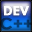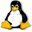Home Latest News Archive Tutorials C++ 2 3 4 Win32 Java OpenGL GLUT OpenGL ES 2 3 4 DirectX VB.NET HTML Photoshop 3dsmax Forums Projects Modelling Links About Us Search Recent Tutorials C / C++ Win32 OpenGL OpenGL ES GLUT## Macros

Tutorials > C++ > Macros

### What are Macros?

Macros are more preprocessor directives that allow you to easily replace code before it is compiled. This allows you to define a common task as a macro. If you decide to change how the action is performed, you only need to change it in one place. A common use of a macro is to define a constant. This is a value that never changes throughout your program.

### How are Macros defined?

Macros are defined in the following way :

#define MACRO_NAME statements

Now, in your program, wherever you place MACRO_NAME, it will be replaced with statements before your program is compiled. An example is as follows :

#define PI 3.14159265

Extra parameters for the macro can be placed in brackets after the MACRO_NAME. Examples of this will be seen further down. Macros are usually named using capital letters.

Contents of main.cpp :

```#include <iostream>
#include <stdlib.h>

using namespace std;
```

Below we define 2 constants. We define CENTURY to have the value of 100 and HELLO to have the value of "Hello World". Notice that we do not add a semi-colon onto the end of the line. If we were to do this, a semi-colon would be added into the generated code, causing syntax errors if not at the end of a statement. These values can now be used multiple times throughout the program. If you wish to change this value at a later stage, only one change needs to be made.

```// Constants
#define CENTURY 100
#define HELLO "Hello World"
```

The example below shows how macros can be defined as a number of statements. Also notice that if you wish to place a macro on multiple lines, you need to place a \ at the end of each line except for the final one. The macro below places 2 carriage returns at the cursors current position.

```#define DOUBLE_BREAK \
cout << endl \
<< endl
```

The PRINTMULT macro below shows how parameters can be used in macros. Brackets are placed afer the macro name. Only an identifier needs to be placed for each parameter. No data types must be given as type checking is not done in the preprocessor phase. This is just for code replacement. In the statements section, you can use the parameter identifiers to place the appropriate value.
Using PRINTMULT(5,4) will output the value of 9 to the console.

```#define PRINTMULT(x,y) \
cout << x * y << endl
```

A problem exists with the above macro. If you specify a more complex expression, errors may occur due to operator precedence.

eg. PRINTMULT(2 + 3, 5 + 2) will be replaced with :

cout << 2 + 3 * 5 + 2 << endl;

Here the 3 * 5 will be calculated before the addition resulting in unexpected results. To fix this, brackets can be placed around the values to ensure that each statement gets processed correctly.

```#define PRINTMULTFIXED(x,y) \
cout << (x) * (y) << endl
```

A # can be placed before a macro parameter in the statements section to replace the identifier with the exact text that is used in the code represented as a string. If you used PRINTMULTTEXT(t, u), #x would output the value "t" and #y would output the value "u".

```#define PRINTMULTTEXT(x,y) \
cout << #x << " * " << \
#y << " = " << x * y << endl
```

The next macro shows how text can be replaced using the # operator.

```#define PRINTPARAGRAPH(text) \
cout << endl << #text \
<< endl << endl

int main()
{
```

Below shows examples of how your constants can be used in our programs.

```	cout << "Century : "
<< CENTURY << endl;

cout << HELLO << endl;
```

Macros consisting of statements can be used in the same way.

```	DOUBLE_BREAK;
cout << "Paragraph" << endl;
DOUBLE_BREAK;
```

Macros with parameters are used by placing the parameters in brackets after the macro name. The macro below outputs a value of 14. This is incorrect as brackets have not been placed to enforce operator precedence.

```	PRINTMULT(5+2, 3+3);
```

The fixed macro is shown below and outputs the correct value of 42.

```	PRINTMULTFIXED(5+2, 3+3);
```

The macro below uses the # operator. Notice that the first time we use the macro, the statements 5+2 and 3+3 are outputted to the console whereas the second utilization yields the values y and z. The actual values you specify in the macro are used.

```	PRINTMULTTEXT(5+2, 3+3);
int y = 3, z = 4;
PRINTMULTTEXT(y, z);
```

The example below shows how the # operator automatically converts the text to a string. Hello World does therefore not have to be in quotes. If you placed quotes below, the text written to the console would also include quotes.

```	PRINTPARAGRAPH(Hello World);

system("pause");

return 0;
}```

### Macros VS Functions

Macros and functions both have their advantages and disadvantages. Macros replace code before compiling. If you have large macros and they are used a number of times, this could result in your final executable being unnecessarily large. When functions are called a slight penalty results due to a system call. In general, you use macros for constants or for extremely small and simple statements. Functions are used in all other cases when a greater amount of complexity is needed.

You should now be able to implement your own macros and you should now understand how macros differ from functions.

Please let me know of any comments you may have : Contact Me

 Source Files :< Tutorial 23 - Passing Parameters Tutorial 25 - Multi-Dimensional Arrays >